# Mathematics - Discrete Mathematics - Conditional Probability

in STEMGeeks6 months ago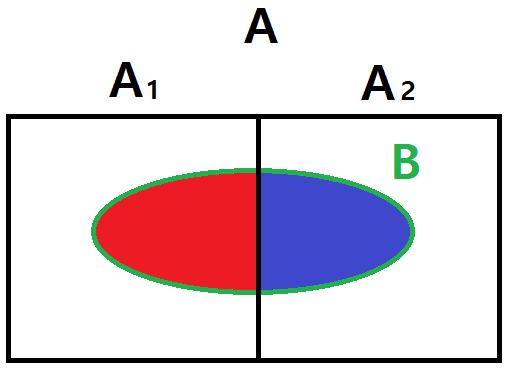[Image 1]

## Introduction

Hey it's a me again @drifter1!

Today we continue with Mathematics, and more specifically the branch of "Discrete Mathematics", in order to get into Conditional Probability.

I highly suggest checking the post on Probability before this one!

So, without further ado, let's get straight into it!

## Conditional Probability

For dependent events, where the occurrence of one affects the outcome of another, a new kind of probability is defined known as conditional probability. The probability of an event A from occurring when an event B has already occurred is denoted as P(A | B), and given by: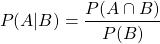Solving for the intersection, results in: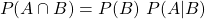In a similar manner, the probability of event B from occurring when A has occurred is: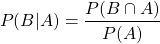If the events A and B are independent then: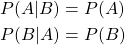### Law of Total Probability

Based on conditional probability, it's possible to calculate the total probability of an event B for any number of disjoint events Ai. The resulting equation is known as the law of total probability: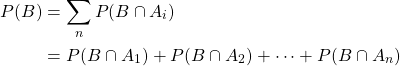Each intersection can also be replaced with the corresponding conditional probability equation yielding: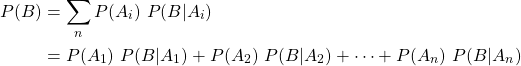### Bayes' Theorem

Let's not forget to mention Bayes' Theorem, which is basically an equation that relates P(A | B) and P(B | A).

It's easy to derive such an equation from the definition of conditional probability: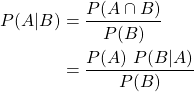Note: Of course commutativity applies for the intersection of A and B.

So, knowing P(A), P(B) and either of the two it's possible to calculate the other using this equation.

## Full-On Example

Consider a bowl is filled with 3 black and 5 white marbles. What's the probability of picking:

• two consecutive black marbles
• black marble followed by white marble
• three consecutive black marbles
• a black marble in the second pick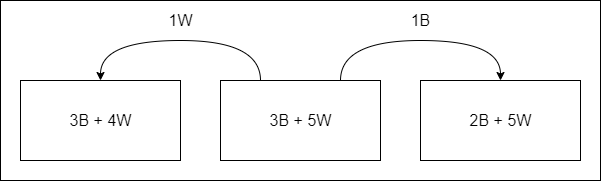The overall number of marbles is 8, and so the probabilities of picking a black and white marble respectively are initially: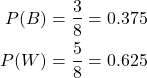### Two consecutive black marbles

After picking a black marble, 7 marbles will be remaining, with only 2 being black. The probability of picking a second black marble, after a black one has already been picked, is thus: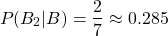And so, the total probability for picking two consecutive black marbles is: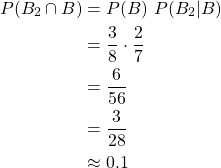### Black marble followed by white marble

After picking a black marble, 5 out of the 7 remaining ones will be white. So, the probability of picking a white one after a black marble is: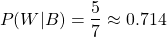As such, the total probability for picking a black marble followed by a white marble is: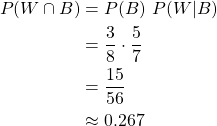Bonus: Of course, the order doesn't matter in this problem, as picking a white one and then a black one has the same probability (it's simply the intersection of B and W in either order). As such, after picking a white one 3 black marbles will remain, giving the same total probability of: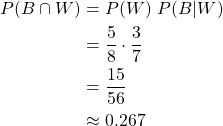### Three consecutive black marbles

After two black marbles have already been picked, picking a third marble has a probability of: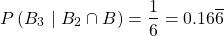as 6 marbles will remain with only 1 being black.

As such, the total probability is: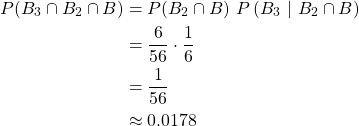In other words, the probability is basically the product: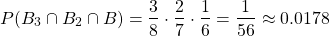as each pick depends on the previous pick.

### Black marble in second pick

This last case is a great example of the law of total probability!

Because it's uncertain what came first, the probability will be a sum of two cases:

• black marble was picked first
• white marble was picked first

So, picking a black marble in the second pick has a probability of: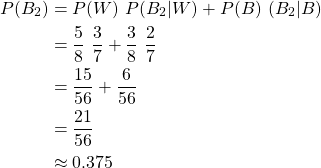## RESOURCES:

### Images

Mathematical equations used in this article, have been generated using quicklatex.

Block diagrams and other visualizations were made using draw.io.

## Final words | Next up

And this is actually it for today's post!

Next time we will get into an overview of Graph Theory...

See ya!Keep on drifting!

Posted with STEMGeeks

Sort:
6 months ago Reveal Comment